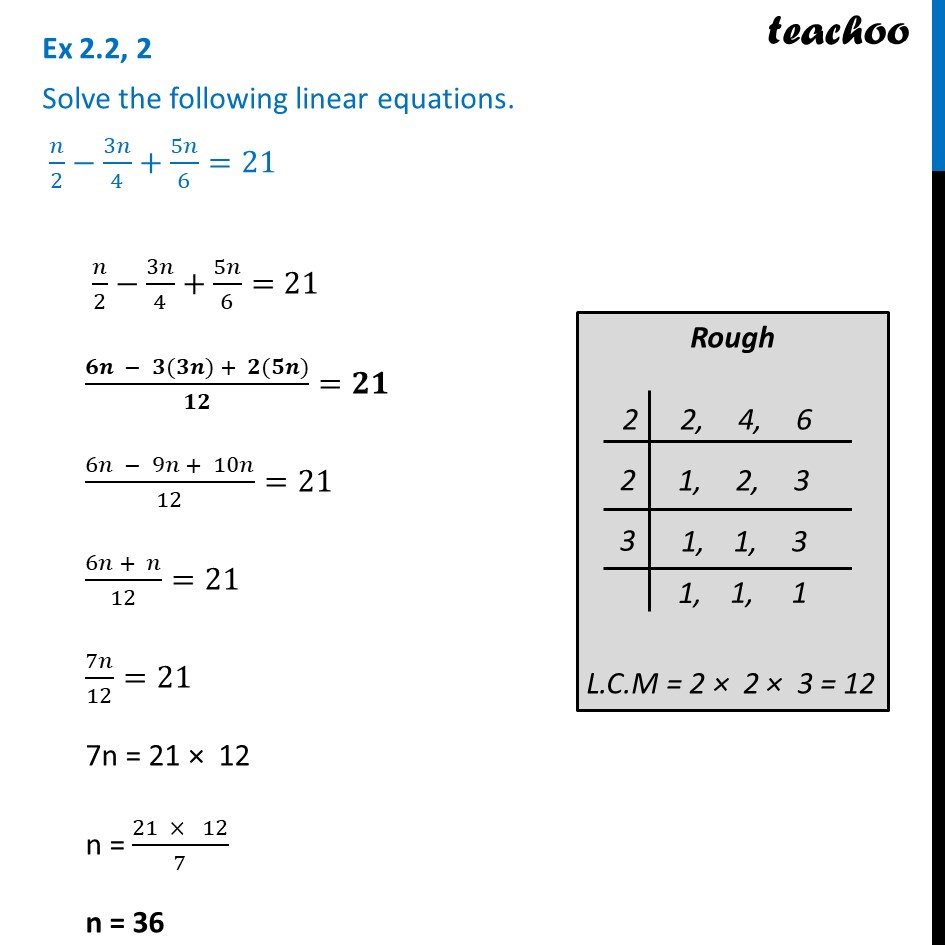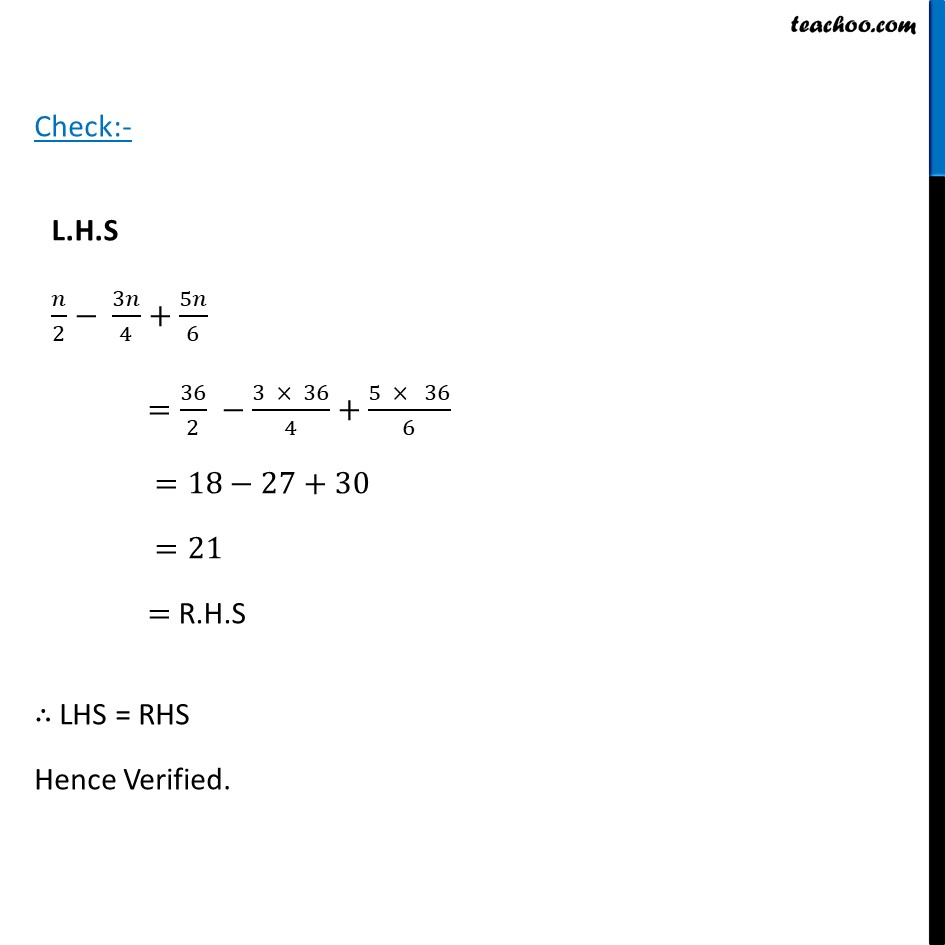Ex 2.2

Chapter 2 Class 8 Linear Equations in One Variable
Serial order wiseLearn in your speed, with individual attention - Teachoo Maths 1-on-1 Class

### Transcript

Ex 2.2, 2 Solve the following linear equations. 𝑛/2−3𝑛/4+5𝑛/6=21 𝑛/2−3𝑛/4+5𝑛/6=21 (𝟔𝒏 − 𝟑(𝟑𝒏) + 𝟐(𝟓𝒏))/𝟏𝟐=𝟐𝟏 (6𝑛 − 9𝑛 + 10𝑛)/12=21 (6𝑛 + 𝑛)/12=21 7𝑛/12=21 7n = 21 × 12 n = (21 × 12)/7 n = 36 L.H.S 𝑛/2− 3𝑛/4+5𝑛/6 =36/2 −(3 × 36)/4+(5 × 36)/6 =18−27+30 =21 = R.H.S Check:- ∴ LHS = RHS Hence Verified.